#Function Repository Resource:

# WelchTest

Compare the means of two observational samples using the Welch test

Contributed by: András Aszódi
 ResourceFunction["WelchTest"][data1,data2] tests whether the two lists of observations data1 and data2 have equal means.

## Details

The Welch test is a modification of the Student t-test that does not require equality of variances.
The Welch test is used to compare the means of two groups of observations (samples) if the samples were drawn from (approximately) normal populations with unequal variances.
ResourceFunction["WelchTest"] returns an association containing the following keys:
 "PValue" p-values "TestStatistic" value of the t-statistic “DegreesOfFreedom" approximate (non-integer) degrees of freedom
ResourceFunction["WelchTest"] takes the following option:
 AlternativeHypothesis "Unequal" the inequality for the alternative hypothesis
With the setting AlternativeHypothesis"Unequal", ResourceFunction["WelchTest"] performs a two-sided Welch test.
Use AlternativeHypothesis"Greater" or AlternativeHypothesis"Less" to perform a one-sided Welch test.

## Examples

### Basic Examples (2)

Two samples with unequal length:

 In:=Perform the two-sided Welch test on the two samples:

 In:=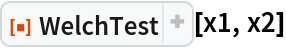Out=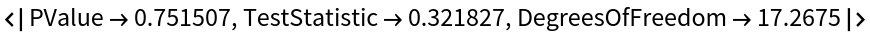### Options (3)

#### AlternativeHypothesis (3)

Two samples with unequal length:

 In:=Test H0:μ1-μ20 versus Ha:μ1-μ2<0:

 In:=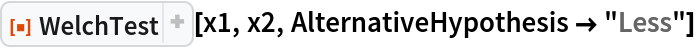Out=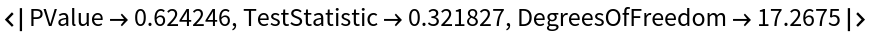Test H0:μ1-μ2<0 versus Ha:μ1-μ20:

 In:=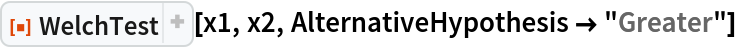Out=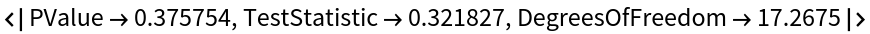Andras Aszodi

## Version History

• 1.0.0 – 08 April 2022